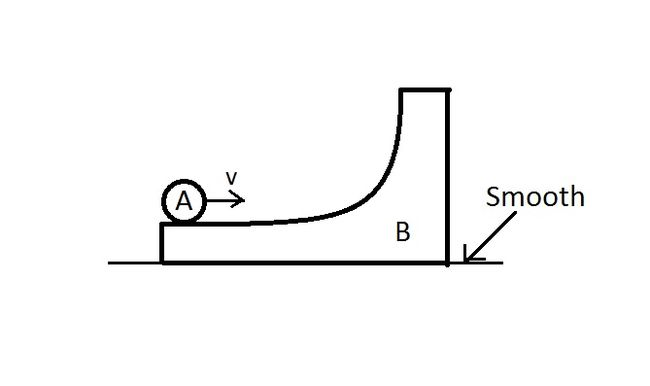# A classical mechanics problem by Arpita KarkeraIn the figure shown a ring $A$ is initially rolling without sliding with a velocity $v$ on the horizontal surface of the body $B$ (of same mass as $A$). All surfaces are smooth. $B$ has no initial velocity. Find the maximum height reached by $A$ on $B$. ($g$ is acceleration due to gravity).

Answer comes out as $\frac{av^{2}}{bg}$ where a and b are coprime integers

What is the value of $a+b$?

×